Courses
Courses for Kids
Free study material
Free LIVE classes
More

# Velocity of Image in Plane Mirror - JEE Important Topic## What Is a Plane Mirror?

Last updated date: 19th Mar 2023
Total views: 61.8k
Views today: 0.41k

When you see yourself in a plane mirror, the image seems inverted. Also, as you move closer, the image moves closer and as you move farther, the image also moves farther. So, from this observation, we can get to the conclusion that the velocity of the image and object might always be opposite. But, what if we are moving parallel to the mirror or the mirror itself is moving in some direction? In these cases, we can’t say the same. So, in this article, we will discuss the velocity of an image in a plane mirror.

## Velocity of Image in a Stationary Plane Mirror

There are different ways in which an object can move in front of a plane mirror. We can’t generalise the velocity of an image with any one case only, so let us see the velocity of image for different cases.

• When an Object is Moving Parallel to the Surface of MirrorImage in Plane Mirror when Object is Moving Parallel to Mirror

When an object moves parallel to the plane mirror, the velocity of the image is the same as that of the object. As the relative distance of the object and the mirror does not change, the image will have velocity parallel to the mirror only and the same as the object.

• When an Object is Moving Perpendicular to the Surface of Mirror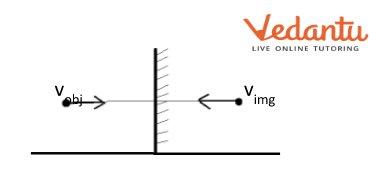Image Formed when Object is Perpendicular

When an object moves perpendicular to the surface of the mirror, the image will have velocity in the direction opposite to the direction of the velocity of the object. However, if the mirror is stationary, the magnitude of velocity of image will be equal to the velocity of object.

Let the velocity of the object be  vobj

Then the velocity of image will be

vimg = - vobj

• When the Velocity of an Object is at Some Angle to the Mirror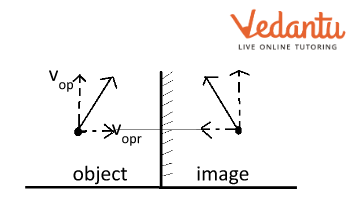Image Formed when Object is at Some Angle

When the velocity of an object is at some angle to the surface of the mirror, we need to resolve the velocity into two perpendicular components such that one component will be parallel to the surface of the mirror and the other will be perpendicular to the surface of the mirror.

If ${{\overset{\to }{\mathop{v}}\,}_{obj}}$ is the velocity of the object then

${{\overset{\to }{\mathop{v}}\,}_{obj}}={{v}_{op}}+{{v}_{opr}}$ where op is the parallel component and opr is the perpendicular

Now we can take the help of the former two cases to write the velocity of the image by taking the parallel component of the object velocity and reversing the perpendicular component.

So let us call the image velocity to be ${{\overset{\to }{\mathop{v}}\,}_{img}}$ then velocity of image in plane mirror formula is given by

${{\overset{\to }{\mathop{v}}\,}_{img}}={{v}_{op}}+(-{{v}_{opr}})$

This is known as plane mirror formula,

## Velocity of Image When Mirror is Moving

We can now calculate velocity of image for any case with the velocity of the object for a stationary mirror. But in case the mirror is moving, some extra effort is needed. When the mirror itself has some velocity, we will consider the mirror as reference and observe the object velocity with respect to the mirror.

•  If mirror is moving parallel to the ground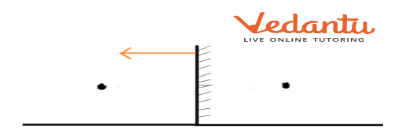Image Formed when Mirror is Moving Parallel to Ground

If vobj is the velocity of object and vm is the velocity of mirror as shown then,

vobj w.r.t mirror will be vobj + vm

so, velocity of image will be –(vobj + vm)

Note that the written image velocity is w.r.t mirror so we need to give the mirror velocity to the image velocity in its original direction.

So, absolute velocity of image will  be =  -vobj vmvm = -(vobj – 2vm)

• If Mirror is Moving Perpendicular to the Ground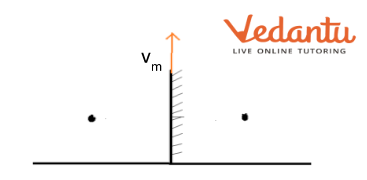Image Formed when Mirror is Moving Perpendicular to Ground

When the mirror will be moving perpendicular to the ground, there will be no change in the velocity of the image as it would be in case of a stationary mirror. This is because at the end, the relative components will cancel out each other and the absolute velocity of the image will be unaffected.

• If Mirror is Moving at an Angle to the Horizontal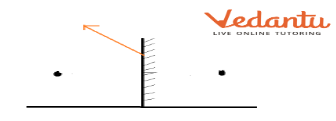Image Formed when Mirror is Moving at an Angle to the Horizontal

If a mirror has a velocity at an angle with respect to the ground, then we need to resolve the mirror velocity into two components i.e., horizontal and vertical components. We can ignore the vertical component as we know from the previous case that it will have no effect on the image velocity. The only remaining component will be the horizontal component. We can find the image velocity as it would be just like as discussed in the first case.

## Velocity of Image When Mirror Surface is at an Angle with the Ground

In the cases we have studied above, the mirror surface was perpendicular with respect to the ground. But in some situations, the mirror surface can be at an angle with the ground.

Let us assume an object approaching a mirror with a horizontal velocity vo and the angle of the mirror surface with the horizontal to be $\theta$.When Mirror Surface is at an Angle with the Ground

In this case, we need to resolve the components of object velocity parallel to the surface of the mirror and perpendicular to the surface, then we can solve for image velocity easily as it is the same as the previous cases.

Velocity component parallel to the surface, ${{v}_{o}}\cos \theta$

Velocity component perpendicular to the surface, ${{v}_{o}}\sin \theta$

Then we can write image velocity as ${{v}_{o}}\cos \theta +(-{{v}_{o}}\sin \theta )$.

Then we can add it vectorially to get the velocity vector of the image.

## Conclusion

In this article, we studied the velocity of an image in different cases. In every case we discussed, the velocity of the image only depends on the relative movement of the object and the mirror. We can always resolve complex cases into simple cases by breaking the velocities into components and then imparting them to image.

In situations involving a combination of two or more of the above-discussed cases, like a moving inclined mirror or both the object velocity and mirror are at an angle with the horizontal, we can break the problems into parts as discussed in the article. Then we can proceed to solve the final problem for the velocity of the image.

## FAQs on Velocity of Image in Plane Mirror - JEE Important Topic

1. If an object is moving at a speed ‘v’ towards the plane mirror, what will be the velocity of the image?

The focal length of a plane mirror is infinity. The plane mirror is a plane reflecting surface in which the incoming rays of light get reflected parallel in opposite directions and they never meet. So they are assumed to meet at an infinite distance. So, if an object is moving at a velocity v towards the mirror, then the velocity of image will be opposite in direction and equal in magnitude to the velocity of the object which will be – v.

2. An object is moving parallel to the surface of a plane mirror. Will the velocity of its image be in the opposite direction?

Yes, in the case of a plane mirror, the image speed and object speed are equal in magnitude and they appear to move towards the reflecting surface, i.e. the mirror.

No, the velocity of the image will not be in the opposite direction. It will be in the same direction as the velocity of the mirror. When any object is moving parallel to the surface of a plane mirror, the image of the object has the same velocity as the object itself.